Select Page

# 12 Science CBSE Answers for MCQ Maths Integrals in English

12 Science CBSE Answers for MCQ Maths Integrals in English to enable students to get Answers in a narrative video format for the specific question.

Expert Teacher provides 12 Science CBSE Answers for MCQ Maths Integrals through Video Answers in English language. This video solution will be useful for students to understand how to write an answer in exam in order to score more marks. This teacher uses a narrative style for a question from Integrals not only to explain the proper method of answering question, but deriving right answer too.

Please find the question below and view the Answer in a narrative video format.

Question:

## Similar Questions from CBSE, 12th Science, Maths, Integrals

Question 1 : Evaluate :(View Answer Video)

Question 2 : Write an antiderivative for the function,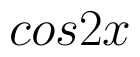using the method of inspection. (View Answer Video)

Question 3 : Evaluate: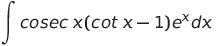(View Answer Video)

Question 4 : Evaluate :(View Answer Video)

Question 5 :(View Answer Video)

### Determinant

Question 1 :  Evaluate the determinants: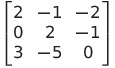. (View Answer Video)

Question 2 :  Evaluate the determinants:. (View Answer Video)

Question 3 : Find the equation of the line joining (3, 1) and (9, 3) using determinants. (View Answer Video)

Question 4 : Evaluate the determinant:. (View Answer Video)

Question 5 : If area of a triangle is 35 sq unit with vertices (2, -6), (5, 4) and (k, 4), then k is, (View Answer Video)

### Vector Algebra

Question 1 : L and M are two points with position vectorsandrespectively. Write the position vectors of a point N which divides the line segment LM in the ratio 2:1 externally.   (View Answer Video)

Question 2 : Find the value of x and y so that the vectorsandare equal. (View Answer Video)

Question 3 : Find the angle between two vectors a and b with magnitudesand 2 respectively, having(View Answer Video)

Question 4 : Find the scalar quantities :
(i)     Work                     (ii)      Force

Question 5 : Find the unit vector in the direction ofifand(View Answer Video)

### Probability

Question 1 : A and B throw a pair of dice alternately. A wins the game if he gets a total of 9 and B wins if he gets a total of 7. If A starts the game, find the probability of winning the game by B.   (View Answer Video)

Question 2 : P speaks truth in 70% of the cases and Q in 80% of the cases. In what percent of cases are they likely to agree in stating the same fact?  (View Answer Video)

Question 3 :  A die is rolled. If E= (1, 3, 5), F(2, 3) and G= {2, 3, 4, 5}, find  (a)(View Answer Video)

Question 4 : If P(A)=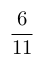, P(B)=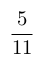and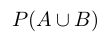=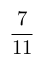, find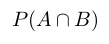. (View Answer Video)

Question 5 : A die is thrown 6 times. If 'getting an odd number' is a 'success', what is the probability of obtaining: